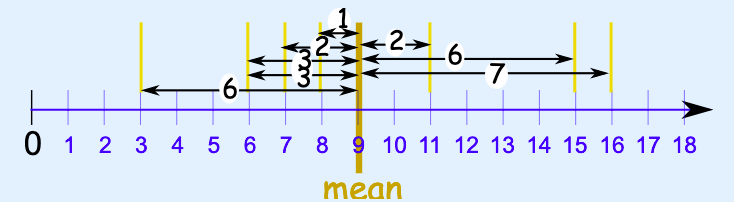# #85 Mean Absolute Deviation (MAD)image taken from https://www.mathsisfun.com/data/mean-deviation.html

### Mean Absolute Deviation (MAD) is how far, on average, all data values are from the middle.

1. Find the mean of the values
2. Find the distance of each value from that mean (subtract the mean from each value, ignore minus signs which is also the absolute value)
3. Then find the mean of those distances